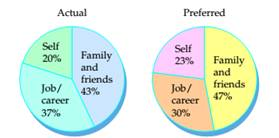# The two circle graphs how surveyed employees actually spend their time and how they would prefer to spend their time. Assume that employees have 112 hours a week that are not spent sleeping. Round answers to the nearest tenth of an hour. (Source: Wall Street Journal Supplement from Families and Work Institute) a. What is the actual number of hours per week that employees spend with family and friends? b. What is the number of hours that employees would prefer to spend on their jobs or careers? c. What is the difference between the number of hours an employee would prefer to spend on him- or herself and the actual amount of time the employee spends on him- or herself?### Mathematical Excursions (MindTap C...

4th Edition
Richard N. Aufmann + 3 others
Publisher: Cengage Learning
ISBN: 9781305965584### Mathematical Excursions (MindTap C...

4th Edition
Richard N. Aufmann + 3 others
Publisher: Cengage Learning
ISBN: 9781305965584

#### Solutions

Chapter
Section
Chapter 9.3, Problem 20ES
Textbook Problem

## The two circle graphs how surveyed employees actually spend their time and how they would prefer to spend their time. Assume that employees have 112 hours a week that are not spent sleeping. Round answers to the nearest tenth of an hour. (Source: Wall Street Journal Supplement from Families and Work Institute)a. What is the actual number of hours per week that employees spend with family and friends?b. What is the number of hours that employees would prefer to spend on their jobs or careers?c. What is the difference between the number of hours an employee would prefer to spend on him- or herself and the actual amount of time the employee spends on him- or herself?Expert Solution

(a)

To determine

To calculate:

The actual number of hours per week that employees spend on their jobs and careers when the two circle graph show how surveyed employees actually spend their time and how they would prefer to spend their time. Assume that employees have 112 hours a week that are not spent sleeping. Round answers to the nearest tenth of an hour. (Source: Wall Street Journal Supplement from Families and Work Institute)

### Explanation of Solution

Given Information:

Two circle graph shows how surveyed employees actually spend their time and how they would prefer spend their time. Assume that employees have 112 hours a week that are not spent sleeping.

Formula used:

Proportion used for percent:

percent100=amountbase

Calculation:

Base is 112, percent for job and percent is 37% ,

The actual number of hours per week that employees sp

Expert Solution

(b)

To determine

To calculate:

The number of hours that employees would prefer to spend on their jobs or careers when the two circle graph show how surveyed employees actually spend their time and how they would prefer to spend their time. Assume that employees have 112 hours a week that are not spent sleeping. Round answers to the nearest tenth of an hour. (Source: Wall Street Journal Supplement from Families and Work Institute)

Expert Solution

(c)

To determine

To calculate:

The difference between the number of hours an employee would prefer to spend on him or herself and the actual of the time the employee spends on him or herself when the two circle graph show how surveyed employees actually spend their time and how they would prefer to spend their time. Assume that employees have 112 hours a week that are not spent sleeping. Round answers to the nearest tenth of an hour. (Source: Wall Street Journal Supplement from Families and Work Institute)

### Want to see the full answer?

Check out a sample textbook solution.See solution

### Want to see this answer and more?

Bartleby provides explanations to thousands of textbook problems written by our experts, many with advanced degrees!

See solution